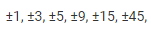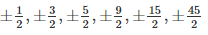Courses

# RD Sharma Solutions Ex-6.5, (Part -1), Factorization Of Polynomials, Class 9, Maths Class 9 Notes | EduRev

## Class 9: RD Sharma Solutions Ex-6.5, (Part -1), Factorization Of Polynomials, Class 9, Maths Class 9 Notes | EduRev

The document RD Sharma Solutions Ex-6.5, (Part -1), Factorization Of Polynomials, Class 9, Maths Class 9 Notes | EduRev is a part of the Class 9 Course RD Sharma Solutions for Class 9 Mathematics.
All you need of Class 9 at this link: Class 9

Q1. x3+6x2+11x+6

Sol:

Given polynomial, f(x) = x3+6x2+11x+6

The constant term in f(x) is 6

The factors of 6 are ±1, ±2, ±3, ±6

Let, x + 1 = 0

⇒ x = -1

Substitute the value of x in f(x)

f(-1) = (−1)3+6(−1)2+11(−1)+6

= -1 + 6 -11 + 6

= 12 – 12

= 0

So, (x + 1) is the factor of f(x)

Similarly, (x + 2) and (x + 3) are also the factors of f(x)

Since, f(x) is a polynomial having a degree 3, it cannot have more than three linear factors.

∴ f(x) = k(x + 1)(x + 2)(x + 3)

⇒ x3+6x2+11x+6 = k(x + 1)(x + 2)(x + 3)

Substitute x = 0 on both the sides

⇒ 0 + 0 + 0 + 6 = k(0 +1)(0 + 2)(0 + 3)

⇒ 6 = k(1*2*3)

⇒ 6 = 6k

⇒ k = 1

Substitute k value in f(x) = k(x + 1)(x + 2)(x + 3)

⇒ f(x) = (1)(x + 1)(x + 2)(x + 3)

⇒ f(x) = (x + 1)(x + 2)(x + 3)

∴ x3+6x2+11x+6 = (x + 1)(x + 2)(x + 3)

Q2. x3+2x2–x–2

Sol:

Given, f(x) = x3+2x2–x–2

The constant term in f(x) is -2

The factors of (-2) are ±1, ±2

Let , x – 1 = 0

⇒ x = 1

Substitute the value of x in f(x)

f(1) = (1)3+2(1)2–1–2

= 1 + 2 – 1 – 2

= 0

Similarly , the other factors (x + 1) and (x + 2) of f(x)

Since, f(x) is a polynomial having a degree 3, it cannot have more than three linear factors.

∴ f(x) = k(x – 1)(x + 2)(x + 1)

x3+2x2–x–2 = k(x – 1)(x + 2)(x + 1)

Substitute x = 0 on both the sides

0 + 0 – 0 – 2 = k(-1)(1)(2)

⇒  – 2 = -2k

⇒  k = 1

Substitute k value in f(x)  = k(x – 1)(x + 2)(x + 1)

f(x) = (1)(x – 1)(x + 2)(x + 1)

⇒ f(x) =  (x – 1)(x + 2)(x + 1)

So, x3+2x2–x–2 = (x – 1)(x + 2)(x + 1)

Q3. x3–6x2+3x+10

Sol:

Let, f(x) = x3–6x2+3x+10

The constant term in f(x) is 10

The factors of 10 are ±1, ±2, ±5, ±10

Let , x + 1 = 0

⇒ x = -1

Substitute the value of x in f(x)

f(-1) = (−1)3–6(−1)2+3(−1)+10

= -1 – 6 – 3 + 10

= 0

Similarly , the other factors (x – 2) and (x – 5) of f(x)

Since, f(x) is a polynomial having a degree 3, it cannot have more than three linear factors.

∴ f(x) = k(x + 1)(x – 2)(x – 5 )

Substitute x = 0 on both sides

⇒ x3–6x2+3x+10 = k(x + 1)(x – 2)(x – 5)

⇒ 0 – 0 + 0 + 10 = k(1)(-2)(-5)

⇒ 10 = k(10)

⇒ k = 1

Substitute k = 1 in f(x) = k(x + 1)(x – 2)(x – 5)

f(x) = (1)(x + 1)(x – 2)(x – 5)

so, x3–6x2+3x+10 = (x + 1)(x – 2)(x – 5)

Q4. x4–7x3+9x2+7x–10

Sol:

Given, f(x) = x4–7x3+9x2+7x–10

The constant term in f(x) is 10

The factors of 10 are ±1, ±2, ±5, ±10

Let , x – 1 = 0

⇒ x = 1

Substitute the value of x in f(x)

f(x) = 14–7(1)3+9(1)2+7(1)–10

= 1 – 7 + 9 + 7 – 10

= 10 – 10

= 0

(x – 1)  is the factor of f(x)

Simarly, the other factors are (x + 1) ,(x – 2) , (x – 5)

Since, f(x) is a polynomial of degree 4. So, it cannot have more than four linear factor.

So, f(x) = k(x – 1)(x + 1)(x – 2)(x – 5)

⇒ x4–7x3+9x2+7x–10 = k(x – 1)(x + 1)(x – 2)(x – 5)

Put x = 0 on both sides

0 – 0 + 0 – 10  =  k(-1)(1)(-2)(-5)

– 10 = k(-10)

⇒ k = 1

Substitute k = 1 in  f(x) = k(x – 1)(x + 1)(x – 2)(x – 5)

f(x) = (1)(x – 1)(x + 1)(x – 2)(x – 5)

= (x – 1)(x + 1)(x – 2)(x – 5)

So, x4–7x3+9x2+7x–10 = (x – 1)(x + 1)(x – 2)(x – 5)

Q5. x4–2x3–7x2+8x+12

Sol:

Given , f(x) = x4–2x3–7x2+8x+12

The constant term f(x) is equal is 12

Tha factors of 12 are ±1, ±2, ±3, ±4, ±6, ±12

Let, x + 1 = 0

⇒ x = -1

Substitute the value of x in f(x)

f(-1) = (−1)4–2(−1)3–7(−1)2+8(−1)+12

= 1 + 2 – 7 – 8 + 12

= 0

So, x + 1 is factor of f(x)

Similarly, (x + 2), (x – 2), (x – 3) are also the factors of f(x)

Since, f(x) is a polynomial of degree 4 , it cannot have more than four linear factors.

⇒ f(x) = k(x + 1)(x + 2)(x – 3)(x – 2)

⇒ x4–2x3–7x2+8x+12 = k(x + 1)(x + 2)(x – 3)(x – 2)

Substitute x = 0 on both sides,

⇒ 0 – 0 – 0 + 12 = k(1)(2)(-2)(-3)

⇒ 12 = k12

⇒ k = 1

Substitute k = 1 in f(x) = k(x – 2)(x + 1)(x + 2)(x – 3)

f(x) = (x – 2)(x + 1)(x + 2)(x – 3)

so, x4–2x3–7x2+8x+12 = (x – 2)(x + 1)(x + 2)(x – 3)

Q6. x4+10x3+35x2+50x+24

Sol:

Given, f(x) = x4+10x3+35x2+50x+24

The constant term in f(x) is equal to 24

The factors of 24 are ±1, ±2, ±3, ±4, ±6, ±8, ±12, ±24

Let, x + 1 = 0

⇒ x = -1

Substitute the value of x in f(x)

f(-1) = (−1)4+10(−1)3+35(−1)2+50(−1)+24

= 1 – 10 + 35 – 50 + 24

= 0

⇒ (x + 1) is the factor of f(x)

Similarly, (x + 2),(x + 3),(x + 4) are also the factors of f(x)

Since, f(x) is a polynomial of degree 4, it cannot have more than four linear factors.

⇒ f(x) = k(x + 1)(x + 2)(x + 3)(x + 4)

⇒ x4+10x3+35x2+50x+24 = k(x + 1)(x + 2)(x + 3)(x + 4)

Substitute x = 0 on both sides

⇒ 0 + 0 + 0 + 0 + 24 = k(1)(2)(3)(4)

⇒ 24 = k(24)

⇒ k = 1

Substitute k = 1 in f(x) = k(x + 1)(x + 2)(x + 3)(x + 4)

f(x) = (1)(x + 1)(x + 2)(x + 3)(x + 4)

f(x) = (x + 1)(x + 2)(x + 3)(x + 4)

hence, x4+10x3+35x2+50x+24 = (x + 1)(x + 2)(x + 3)(x + 4)

Q7.  2x4–7x3–13x2+63x–45

Sol :

Given, f(x) = 2x4–7x3–13x2+63x–45

The factors of constant term -45 are ±1, ±3, ±5, ±9, ±15, ±45

The factors of the coefficient of  x4 is 2. Hence possible rational roots of f(x) areLet, x – 1 = 0

⇒ x= 1

f(1) = 2(1)4–7(1)3–13(1)2+63(1)–45

= 2 – 7 – 13 + 63 – 45

= 0

Let, x – 3 = 0

⇒ x = 3

f(3) = 2(3)4–7(3)3–13(3)2+63(3)–45

= 162 – 189 – 117 + 189 – 45

= 0

So, (x – 1) and (x – 3) are the roots of f(x)

⇒ x2 – 4x + 3 is the factor of f(x)

Divide f(x) with x2 – 4x + 3 to get other three factors

By long division,

2x2 + x – 15

x2  – 4x + 3    2x4 – 7x3 – 13x + 63x – 45

2x – 8x + 6x2

(-)      (+)        (-)

x3   – 19x + 63x

x3     – 4x2     + 3x

(-)        (+)          (-)

– 15x2 + 60x – 45

(+)           (-)         (+)

0

⇒  2x4–7x3–13x2+63x–45 = (x2 – 4x + 3)(2x2 + x – 15)

⇒ 2x4–7x3–13x2+63x–45 = (x – 1) (x – 3)(2x2 + x – 15)

Now,

2x2 + x – 15 = 2x2+ 6x – 5x  – 15

= 2x(x + 3) – 5 (x + 3)

= (2x – 5) (x + 3)

So, 2x4–7x3–13x2+63x–45 = (x – 1)(x – 3)(x + 3)(2x – 5)

Q8. 3x3−x2–3x+1

Sol :

Given , f(x) = 3x3−x2–3x+1

The factors of constant term 1 is ±1

The factors of the coefficient of x2 = 3

The possible rational roots are ±1 ,1/3

Let, x – 1 = 0

⇒ x = 1

f(1) = 3(1)3−(1)2–3(1)+1

= 3 – 1 – 3 + 1

= 0

So, x – 1 is tha factor of f(x)

Now, divide f(x) with (x – 1) to get other factors

By long division method,

3x2 + 2x – 1

x – 1   3x3 – x– 3x + 1

3x3 – x2

(-)       (+)

2x2 – 3x

2x2 – 2x

(-)       (+)

-x + 1

-x + 1

(+)  (-)

0

⇒ 3x3−x2–3x+1 = (x – 1)( 3x2 + 2x – 1)

Now,

3x2 + 2x – 1 = 3x2 + 3x – x  – 1

= 3x(x + 1) -1(x + 1)

= (3x – 1)(x + 1)

Hence , 3x3−x2–3x+1 = (x – 1) (3x – 1)(x + 1)

Q9. x3−23x2+142x–120

Sol :

Let, f(x) = x3−23x2+142x–120

The constant term in f(x) is -120

The factors of -120 are ±1, ±2, ±3, ±4, ±5, ±6, ±8, ±10, ±12, ±15, ±20, ±24, ±30, ±40, ±60, ±120

Let, x – 1 = 0

⇒ x = 1

f(1) = (1)3−23(1)2+142(1)–120

= 1 – 23  + 142 – 120

= 0

So, (x – 1) is the factor of f(x)

Now, divide f(x) with (x – 1) to get other factors

By long division,

x2 – 22x + 120

x – 1   x3 – 23x2 + 142x – 120

x3 –   x2

(-)    (+)

-22x2 + 142x

-22x2  + 22x

(+)            (-)

120x – 120

120x – 120

(-)           (+)

0

⇒ x3 – 23x2 + 142x – 120 = (x  – 1) (x2 – 22x + 120)

Now,

x2 – 22x + 120 = x2 – 10x – 12x  + 120

= x( x – 10) – 12( x – 10)

= (x – 10) (x – 12)

Hence, x3 – 23x2 + 142x – 120 = (x – 1) (x – 10) (x – 12

The document RD Sharma Solutions Ex-6.5, (Part -1), Factorization Of Polynomials, Class 9, Maths Class 9 Notes | EduRev is a part of the Class 9 Course RD Sharma Solutions for Class 9 Mathematics.
All you need of Class 9 at this link: Class 9Use Code STAYHOME200 and get INR 200 additional OFF Use Coupon Code
All Tests, Videos & Notes of Class 9: Class 9

### Up next### Top Courses for Class 9## RD Sharma Solutions for Class 9 Mathematics

91 docs

### Up next### Top Courses for Class 9Track your progress, build streaks, highlight & save important lessons and more!

,

,

,

,

,

,

,

,

,

,

,

,

,

,

,

,

,

,

,

,

,

,

,

,

,

,

,

,

,

,

,

,

,

;